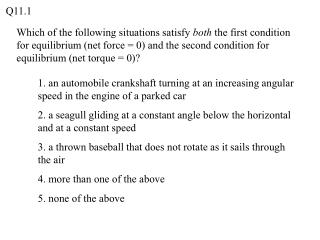DownloadDownload Presentation1. an automobile crankshaft turning at an increasing angular speed in the engine of a parked car

1. an automobile crankshaft turning at an increasing angular speed in the engine of a parked car

Télécharger la présentation1. an automobile crankshaft turning at an increasing angular speed in the engine of a parked car

- - - - - - - - - - - - - - - - - - - - - - - - - - - E N D - - - - - - - - - - - - - - - - - - - - - - - - - - -
Presentation Transcript

1. Q11.1 Which of the following situations satisfy both the first condition for equilibrium (net force = 0) and the second condition for equilibrium (net torque = 0)? 1. an automobile crankshaft turning at an increasing angular speed in the engine of a parked car 2. a seagull gliding at a constant angle below the horizontal and at a constant speed 3. a thrown baseball that does not rotate as it sails through the air 4. more than one of the above 5. none of the above

2. A11.1 Which of the following situations satisfy both the first condition for equilibrium (net force = 0) and the second condition for equilibrium (net torque = 0)? 1. an automobile crankshaft turning at an increasing angular speed in the engine of a parked car 2. a seagull gliding at a constant angle below the horizontal and at a constant speed 3. a thrown baseball that does not rotate as it sails through the air 4. more than one of the above 5. none of the above

3. Q11.2 A massless rod of length L is suspended from the ceiling by a string attached to the center of the rod. A sphere of mass M is suspended from the left-hand end of the rod. Where should a second sphere of mass 2M be suspended so that the rod remains horizontal? 1. at x = 2L/3 2. at x = 3L/4 3. at x = 4L/5 4. at x = 3L/5 5. none of the above

4. A11.2 A massless rod of length L is suspended from the ceiling by a string attached to the center of the rod. A sphere of mass M is suspended from the left-hand end of the rod. Where should a second sphere of mass 2M be suspended so that the rod remains horizontal? 1. at x = 2L/3 2. at x = 3L/4 3. at x = 4L/5 4. at x = 3L/5 5. none of the above

5. Q11.3 A metal advertising sign (weight w) is suspended from the end of a massless rod of length L. The rod is supported at one end by a hinge at point P and at the other end by a cable at an angle q from the horizontal. What is the tension in the cable? 1. T = w sin q 2. T = w cos q 3. T = w/(sin q) 4. T = w/(cos q) 5. none of the above

6. A11.3 A metal advertising sign (weight w) is suspended from the end of a massless rod of length L. The rod is supported at one end by a hinge at point P and at the other end by a cable at an angle q from the horizontal. What is the tension in the cable? 1. T = w sin q 2. T = w cos q 3. T = w/(sin q) 4. T = w/(cos q) 5. none of the above

7. Q11.4 When an airplane is flying at a constant altitude, three vertical forces act on it — the weight w, an upward lift force L exerted by the wing, and a downward force T exerted by the tail.In equilibrium, what are the magnitudes L and T? 1. L = w, T = 0 2. L = w(1 + a/b), T = w(a/b) 3. L = w(1 – a/b), T = w(a/b) 4. L = w(a/b), T = w(1 + a/b) 5. L = w(a/b), T = w(1 – a/b)

8. A11.4 When an airplane is flying at a constant altitude, three vertical forces act on it — the weight w, an upward lift force L exerted by the wing, and a downward force T exerted by the tail.In equilibrium, what are the magnitudes L and T? 1. L = w, T = 0 2. L = w(1 + a/b), T = w(a/b) 3. L = w(1 – a/b), T = w(a/b) 4. L = w(a/b), T = w(1 + a/b) 5. L = w(a/b), T = w(1 – a/b)

9. Q11.5 Two rods — one of length L, the other of length L/2 — are made of the same kind of steel and have the same diameter. A force of magnitude F is applied to the end of each rod as shown. Compared to the rod of length L, the rod of length L/2 has 1. more stress and more strain 2. the same stress and more strain 3. the same stress and less strain 4. less stress and the same strain 5. the same stress and the same strain

10. A11.5 Two rods — one of length L, the other of length L/2 — are made of the same kind of steel and have the same diameter. A force of magnitude F is applied to the end of each rod as shown. Compared to the rod of length L, the rod of length L/2 has 1. more stress and more strain 2. the same stress and more strain 3. the same stress and less strain 4. less stress and the same strain 5. the same stress and the same strain